# Distance Speed and Time D Speed distance in

• Slides: 7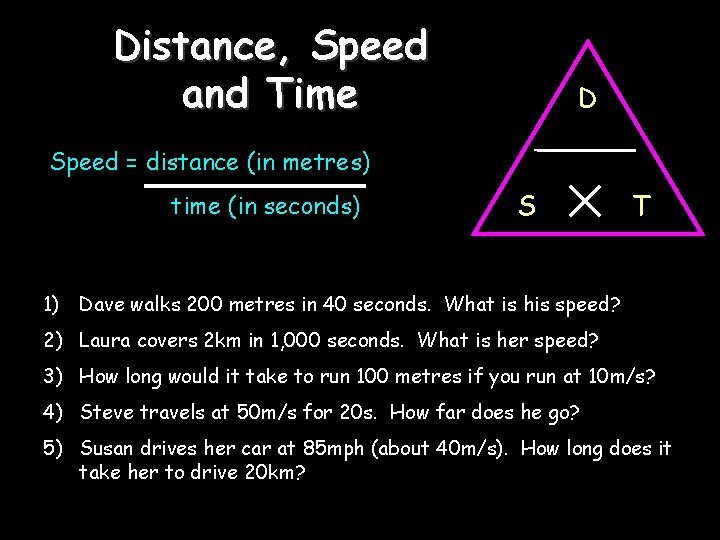Distance, Speed and Time D Speed = distance (in metres) time (in seconds) S T 1) Dave walks 200 metres in 40 seconds. What is his speed? 2) Laura covers 2 km in 1, 000 seconds. What is her speed? 3) How long would it take to run 100 metres if you run at 10 m/s? 4) Steve travels at 50 m/s for 20 s. How far does he go? 5) Susan drives her car at 85 mph (about 40 m/s). How long does it take her to drive 20 km?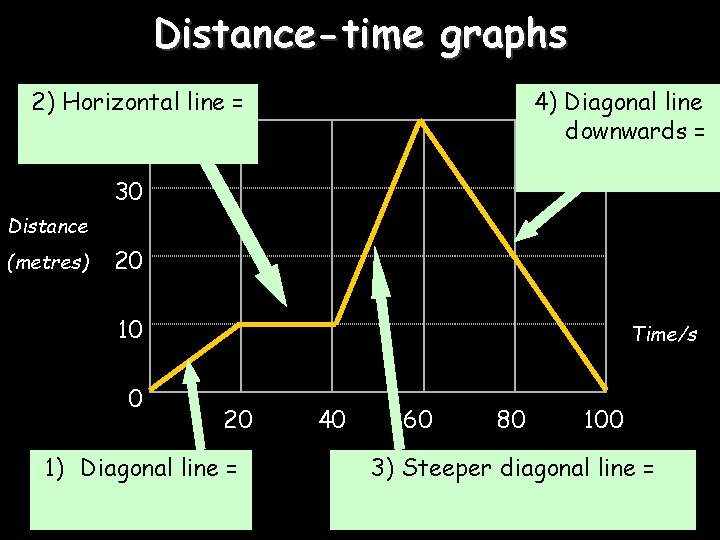Distance-time graphs 2) Horizontal line = 40 4) Diagonal line downwards = 30 Distance (metres) 20 10 0 Time/s 20 1) Diagonal line = 40 60 80 100 3) Steeper diagonal line =40 Distance (metres) 30 20 10 0 Time/s 20 40 60 80 100 1) What is the speed during the first 20 seconds? 2) How far is the object from the start after 60 seconds? 3) What is the speed during the last 40 seconds? 4) When was the object travelling the fastest?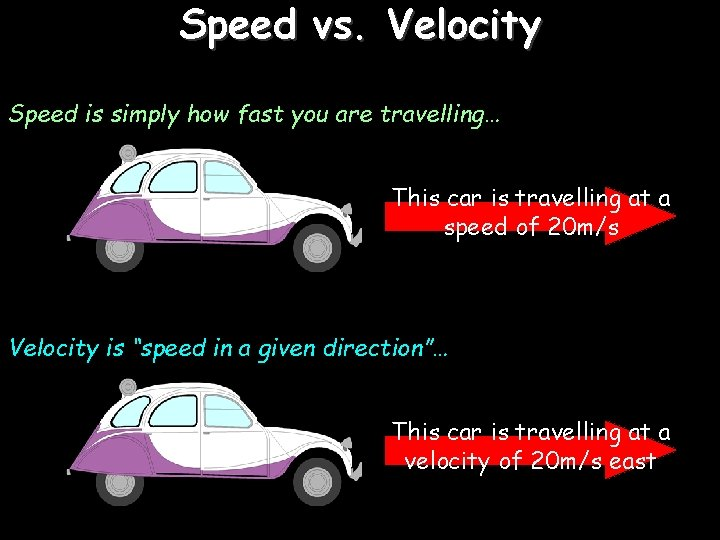Speed vs. Velocity Speed is simply how fast you are travelling… This car is travelling at a speed of 20 m/s Velocity is “speed in a given direction”… This car is travelling at a velocity of 20 m/s east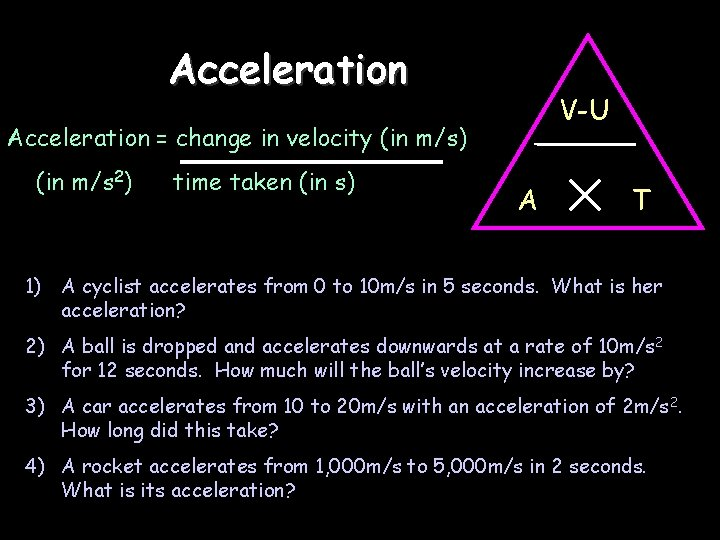Acceleration V-U Acceleration = change in velocity (in m/s) (in m/s 2) time taken (in s) A T 1) A cyclist accelerates from 0 to 10 m/s in 5 seconds. What is her acceleration? 2) A ball is dropped and accelerates downwards at a rate of 10 m/s 2 for 12 seconds. How much will the ball’s velocity increase by? 3) A car accelerates from 10 to 20 m/s with an acceleration of 2 m/s 2. How long did this take? 4) A rocket accelerates from 1, 000 m/s to 5, 000 m/s in 2 seconds. What is its acceleration?Velocity-time graphs 1) Upwards line = 80 Velocity m/s 4) Downward line = 60 40 20 0 10 2) Horizontal line = 20 30 40 50 3) Upwards line = T/s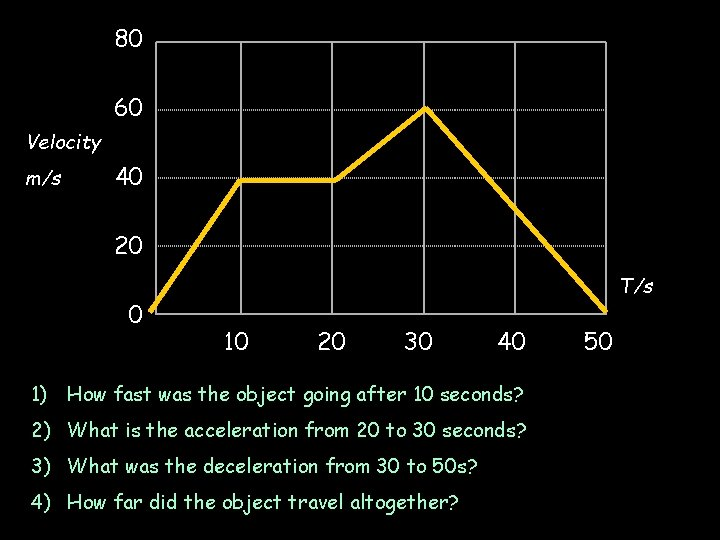80 60 Velocity m/s 40 20 0 T/s 10 20 30 40 1) How fast was the object going after 10 seconds? 2) What is the acceleration from 20 to 30 seconds? 3) What was the deceleration from 30 to 50 s? 4) How far did the object travel altogether? 50